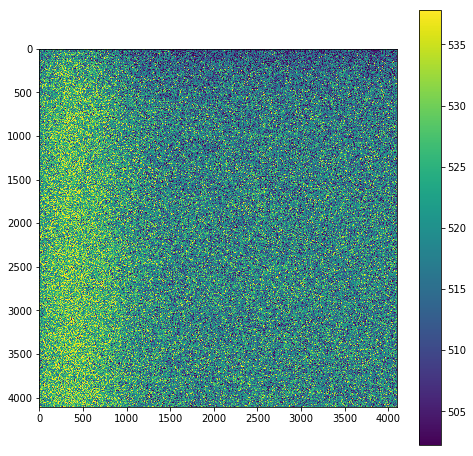# Image Reduction Module¶

Jupyter Notebook Author: Kishalay De & Cameron Hummels

This is a Jupyter notebook lesson taken from the GROWTH Winter School 2018. For other lessons and their accompanying lectures, please see: http://growth.caltech.edu/growth-astro-school-2018-resources.html

## Objective¶

Process raw images from a visible wavelength telescope and make them ready for photometric analysis

## Key steps¶

• Reduce raw images by applying biases & flats
• Align consecutive images

## Required dependencies¶

See GROWTH school webpage for detailed instructions on how to install these modules and packages. Nominally, you should be able to install the python modules with pip install <module>. The external astromatic packages are easiest installed using package managers (e.g., rpm, apt-get).

• python 3
• astropy
• numpy
• matplotlib
• astroscrappy
• pyregion

### External packages¶

In :
#import some useful modules
from astropy.io import fits
import matplotlib.pyplot as plt
import glob
import os
from astropy.stats import sigma_clipped_stats
import numpy as np
import subprocess
import astroscrappy
import pyregion
import shutil


## Test dependencies¶

In order for this jupyter notebook to function correctly, we must have some external software installed, as described above. The following step assures that these are installed properly before getting to the rest of the content of this lesson.

In :
dependencies = ['SWarp', 'sextractor']

def test_dependency(dep):
try:
print("%s is installed properly. OK" % dep)
return 1
except ImportError:
print("===%s IS NOT YET INSTALLED PROPERLY===" % dep)
return 0

i = 0
for dep in dependencies:
i += test_dependency(dep)
print("\n%i out of %i dependencies installed properly." % (i, len(dependencies)))
if i != len(dependencies):
print("Please correctly install these programs before continuing.")
else:

SWarp is installed properly. OK
sextractor is installed properly. OK

2 out of 2 dependencies installed properly.


## Define where data live¶

The various directories where data live are defined here for use later in this notebook

In :
curpath = os.path.abspath('.')                  # top level directory
dataFolder = os.path.join(curpath, 'data')      # data dir
biasFolder = os.path.join(dataFolder, 'bias')   # biases
flatFolder = os.path.join(dataFolder, 'flats')  # flats
sciFolder = os.path.join(dataFolder, 'science') # science data
procFolder = os.path.join(curpath, 'processing')# processing directory
if not os.path.isdir(procFolder):
os.mkdir(procFolder)
else:
for f in os.listdir(procFolder):
os.remove(os.path.join(procFolder,f)) #clear the processing folder from previous iterations

In :
os.chdir(sciFolder)
fileList = glob.glob('*.fits')
os.chdir(curpath)
procList = [os.path.join(procFolder, file) for file in fileList]
sciList = [os.path.join(sciFolder, file) for file in fileList]


## Data description¶

The image data received from the telescope is in the form of a FITS file containing a 2D array of counts that represent the brightness distribution on the sky as seen by the detector. We have to remove the effects of the telescope optical chain from this image to get the true brightness distribution on the sky. There are are a few different steps involved here. We will call this image distribution $I(x,y)$ as a function of x, y coordinates.

• Bias correction: The detector image comes with an electronic offset introduced by the voltages applied to the the semiconductor-based detector. We have to first remove this offset to get real counts received by the detector. Let's call this offset $B(x,y)$. Thw way to measure these offsets is to record images with zero exposure time, so that the recorded image reflects the intrinsic offsets in the detector (since the detector does not receive any photons if the exposure time is zero).

## Creating a bias frame¶

We'll start this module by creating a master bias frame to be subtracted from each of the science and flat images. The idea is to use a median combination of multiple bias frames to get rid of transient features like cosmic rays in each of the individual bias frames, and create a 'Master bias' frame.

In :
biasList = glob.glob(os.path.join(biasFolder,'*fits'))

numBiasFiles = len(biasList)
print('Found %d bias files'%numBiasFiles)

#Let's load all the files into a numpy array
#This is a 3D array with each element representing a 4096 x 4108 pixel array corresponding to each input image
biasImages = np.zeros((4108, 4096, numBiasFiles))

for i in range(numBiasFiles):
biasImages[:,:,i] = fits.open(biasList[i]).data

#Let's plot up a single bias frame
plt.figure(figsize=(8,8))
mean, median, std = sigma_clipped_stats(biasImages[:,:,0])
plt.figure(figsize=(8,8))
#set the scale of the image based on its statistics
plt.imshow(biasImages[:,:,0], vmin = median - 2*std, vmax = median + 2*std)
plt.colorbar()
plt.show()

#Now creating the master bias frame by doing a median combination for each pixel in the list of images
masterBias = np.median(biasImages, axis=2)

Found 3 bias files

<Figure size 576x576 with 0 Axes>Now plot the master bias frame and check what it looks like

In :
#Lets plot the bias image
mean, median, std = sigma_clipped_stats(masterBias)
plt.figure(figsize=(8,8))
#set the scale of the image based on its statistics
plt.imshow(masterBias, vmin = median - 2*std, vmax = median + 2*std)
plt.colorbar()
plt.show()